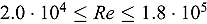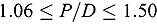This paper is aimed at the application of computational fluid dynamics (CFD) calculations for lateral flow modeling in rod bundles of Russian-type pressurized water reactors with hexagonal fuel rod lattice by subchannel analysis under a constant temperature. The subchannel code SUBCAL and CFD code ANSYS Fluent with the Reynolds stress turbulence model, which is capable of solving the anisotropic flow present in rod bundles, are used. Both methods are compared in terms of calculations in rod bundles. The literature review of available experiments of rod bundles suitable for CFD calculation validation follows. This paper describes the created CFD models on a triangular lattice, which are subsequently validated on selected experimental data in a wide range of Reynolds numbersand geometry (pitch-to–rod diameter ratio)together with mesh sensitivity analysis. The main part of this work is to develop a new equation for the lateral flow resistance coefficient for the subchannel code based on CFD calculations. Within these calculations, the turbulent mixing coefficient β for hydraulically smooth rod bundles, which is related to the geometry, and the momentum-energy transfer analogy correction factor ε are also evaluated and for which the equation is subsequently proposed.